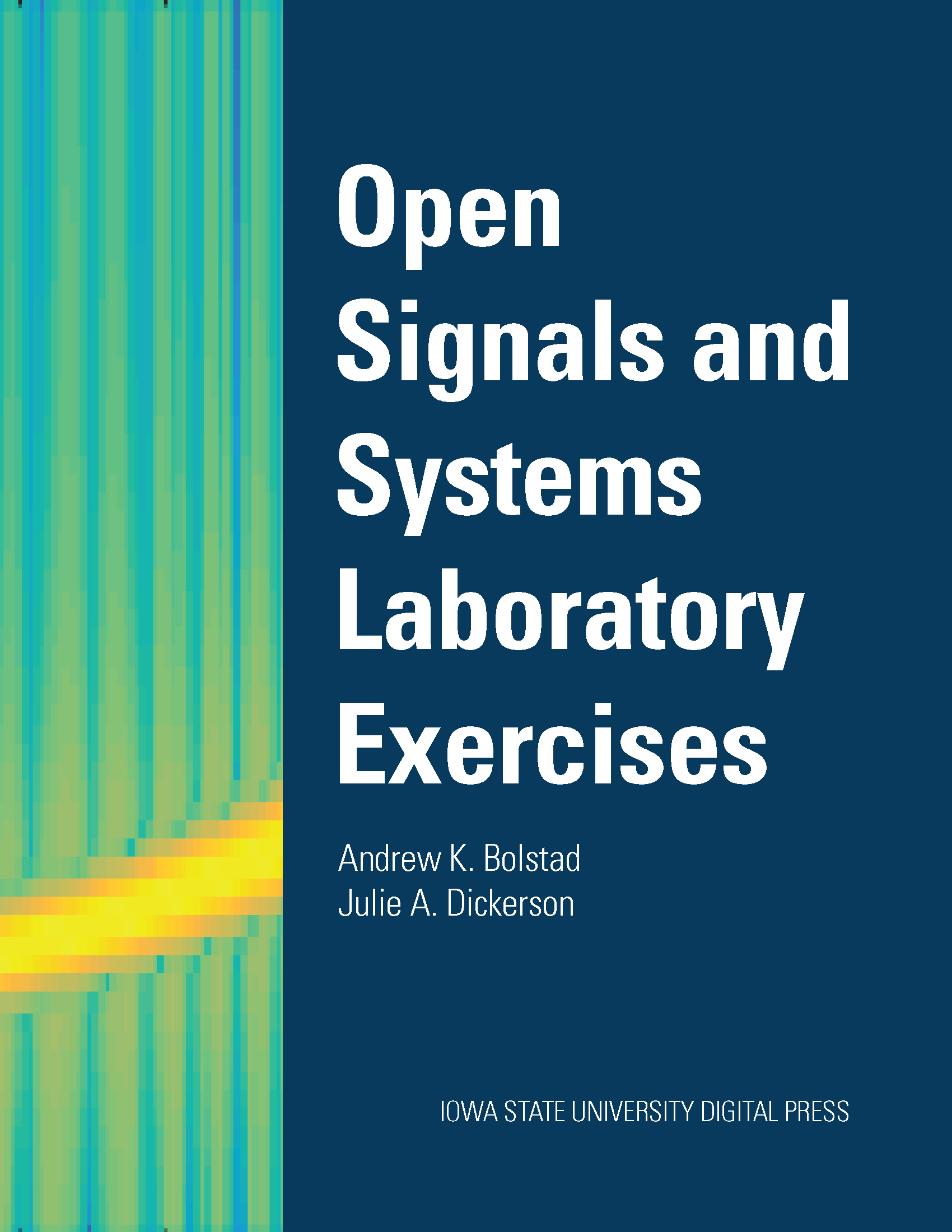## MATLAB Basics (PDF)

### Andrew K. Bolstad & Julie A. Dickerson

This chapter is part of: Bolstad A. & Dickerson J. 2021. Open Signals and Systems Laboratory Exercises

Description
The first lab exercise introduces students to the MATLAB environment and language with an emphasis on signal processing applications. Students solve a system of equations, manipulate complex numbers, vectorize an equation, and manipulate sound and image files.
• Details